## Friday, 15 July 2011

### Irrationality

Rational and irrational numbers are an interesting topic and yet not all the problems in the related field has been solved fully. I shall now introduce some basic properties of it.

1) Group as an algebraic structure have the following properties
For a group G there exist a function, say * here.
(Just recall that the function maps a single element from a set to a unique element in co-domain.)
-Closure. i.e., if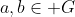, then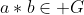.
-Associativity: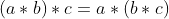-Existance of identity: exist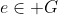identity  such that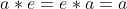, it's often written as 1 (Since 1 is the identity of real/rational # under multiplication.)
-Existance of inverse element: for every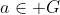, exist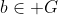(it can be a) such that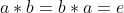. (However there are theorem saying that if ab = e, then ba = e). We say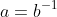. (NEVER a = 1/b)

Example: non-zero Rational number with multiplication is a group, identity 1.

2) Rational numbers can be written in forms of p/q where p is integer and q is natrual number. More precisely rational numbers have their own unique expressions if (|p|,|q|) = 1.
Inversely irrational numbers can't be expressed in fractions, this gives us the fundamental way to show that a number is irrational.

Example 2: Prove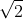is irrational.
Classic question. Assume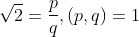.
By squaring both sides and change of term, we have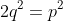.
Then following logic finishes the prove by contradiction.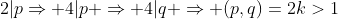.

Now we will introduce another method to show irrationality.
Assume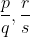are two simpliest distinct rationals with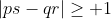, then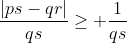so that we can write a real number in a series of fractions. More importantly, irrational numbers can't be written in finite numbers of fractions.

Corollary: Fractions, in decimal, are recurring.
Prove: Consider prime p, we are going to prove 1/p is recurring.
Consider Fermat's little theorem,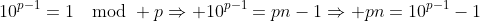.
Therefore p is a divisor of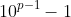.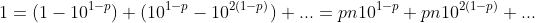Therefore 1/p is recurring.

Note that recurring period may not be p-1 if it can be further simplified, but the general trend is rising.
Proof: For every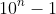, there exist finite prime factors so that they are generally used up, so that larger primes are GENERALLY mapped to higher n.

Then if the recurring decimal is infinity, it is irrational. That gives the idea to prove a number being irrational.

Example 3: Prove e is irrational.
Consider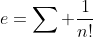, set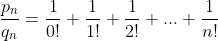, it's a fraction with denominator n!., it follows that we can't express e in finite number of fractions, so we have completed the proof.
Another approach is directly combining terms by definition, since the expressed forms in simpliest terms* monotonically increase, the sum has a infinitely large denominator, hence irrational.

4) Back to out main concern, we would like to show some properties of irrational numbers.
- Irrational numbers do not perform closure under most elementary functions.
Example 4: Proof the existance of infinite pairs of (x,y) where x,y are irrational and x+y, xy are rational. More precisely, show that for every irrational x show that there's infinitely many y satisfying the condition.
Proof: Letwhere r is rational.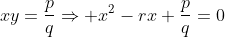, with root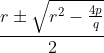by adjusting r obviously there exist many r such that the outcoming x is irrational. Our prove is then completed.

5) Show that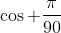is irrational.
This is a bit tricky. We show it by De Morive's theorem.
By expanding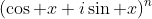, we find that cos nx are expressed in terms of P(cos x), where P is a polynomial of rational coefficients. Therefore irrationality of cos nx implies irrationality of cos x. Consider the irrationality of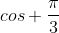, the proof is completed.

Exercise:
1) Show that (Z,+) is a group.
2) Show that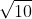is irrational.
3) Show thatis irrational by showing that it can't be written in finite fractional.
4) Show that irrational numbers can be written in continued fraction of infinite length.
5) Show that 37 is a factor of 999 considering 1/37.
6) Provide a full mechanism such that there's infinite r to satisfy the considition "x,y, irrational and x+y, xy rational".
7) Discuss the countability of irrational numbers.
8) Discuss the countability of irrational pairs (x,y) such that x+y and xy are rational.
9) If x,y are irrational while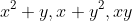is rational. Find all possible x+y with proof.
10) Show that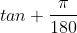is irrational.
11) For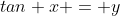, if y is rational, prove the primitive root of x (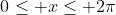) is irrational, unless it is trivially rational (e.g. tan 0 = 0).
12) Show that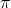is irrational.
13) If x,y are rational numbers with x>y, show that there exist rational numbers a,b and irrational number c that x>a>c>b>y. Hence prove real number is dense.

Extra infomation: we prove the transcendency of a number by similar method but stricter bounding. You can visit the page talking about Liouville's numbers. Then finish this question:
CGMO 2007 day 2 Q3
If a,b,c are integers that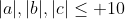and define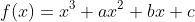satisfying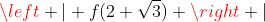is smaller than 0.0001, prove that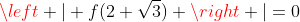.